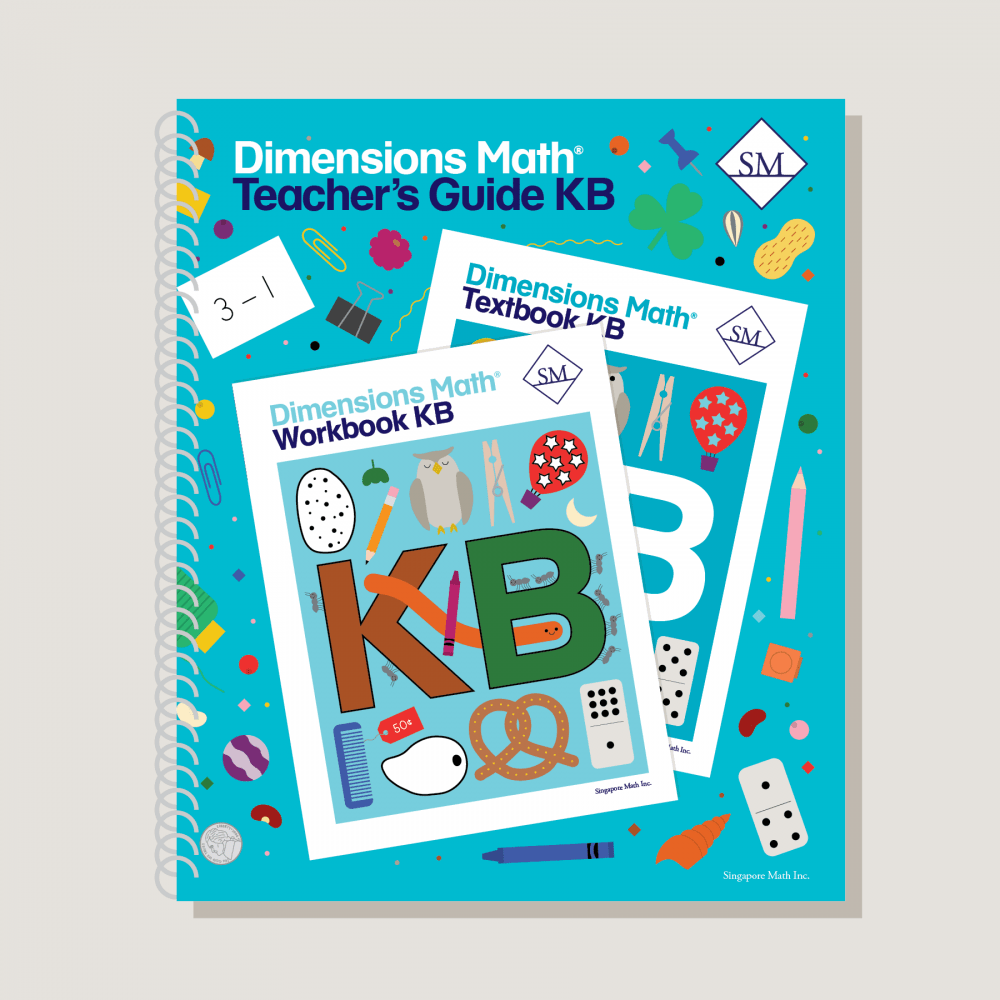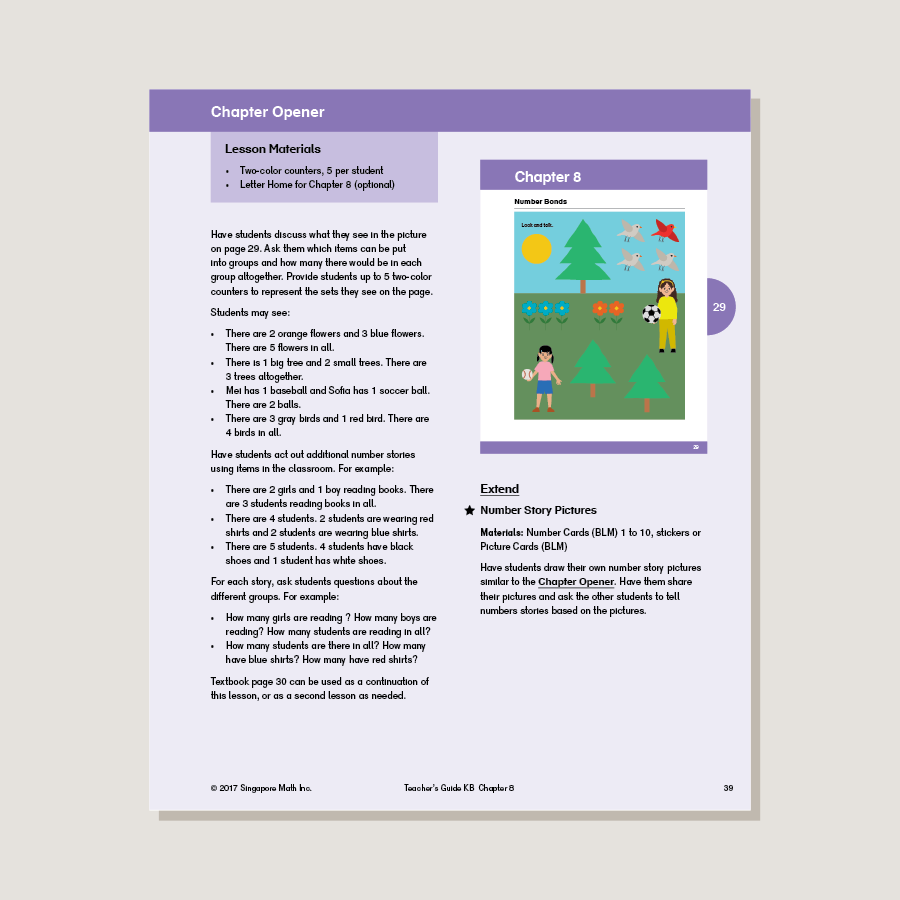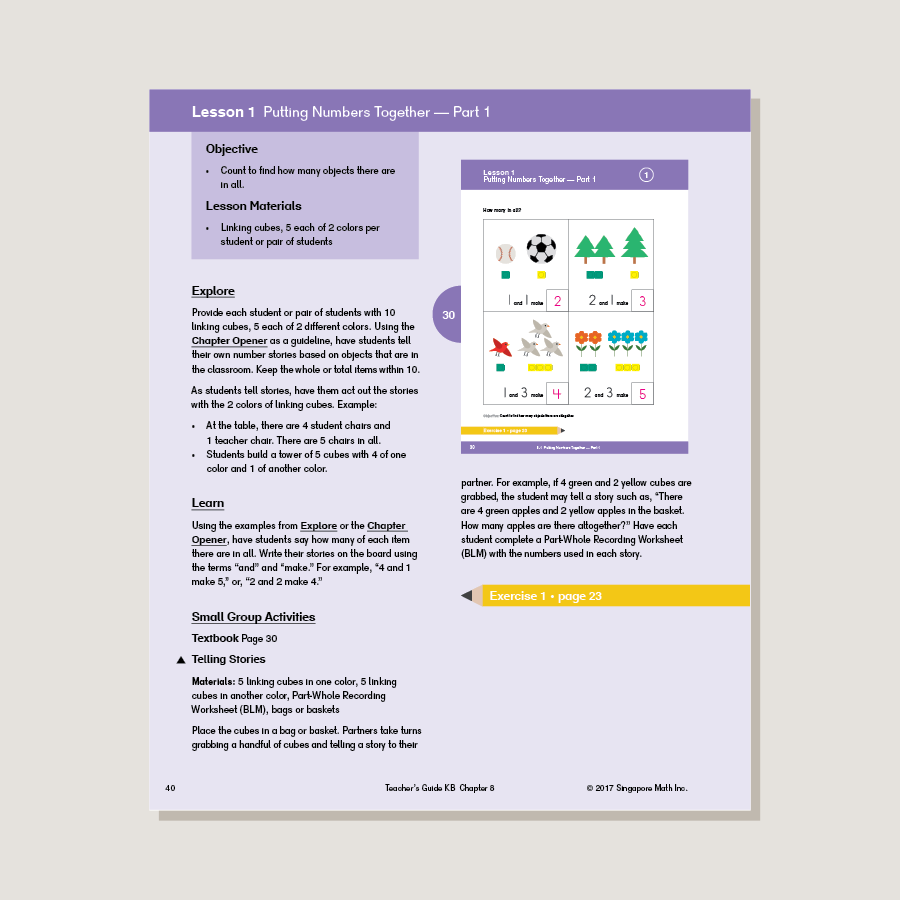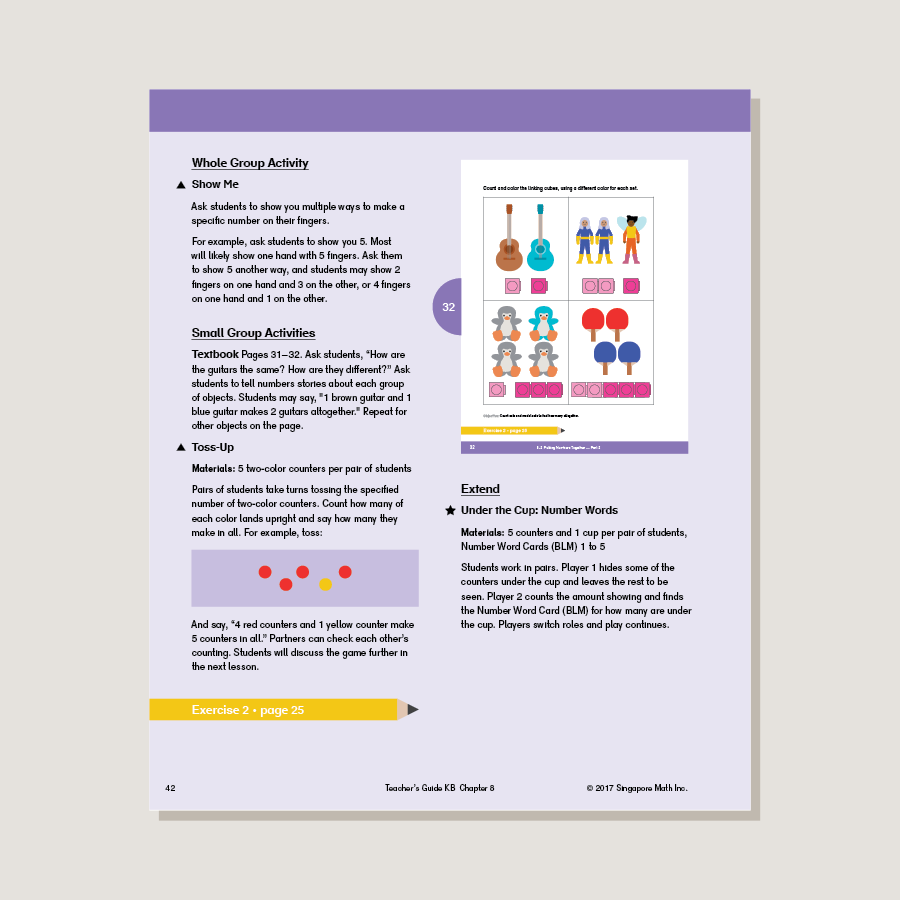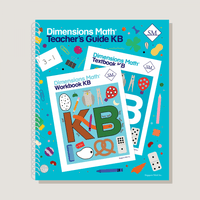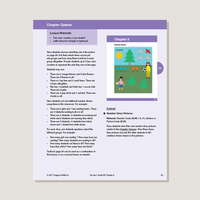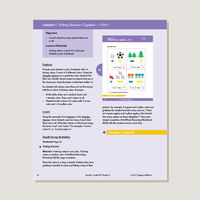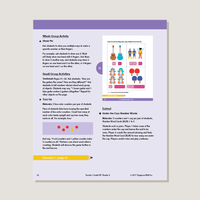# Dimensions Math Teacher's Guide KB

\$31.50

Teacher’s guides are a comprehensive resource for achieving lesson objectives. They include background information, detailed lesson plans, helpful suggestions, and activities. They help educators understand the purpose of each lesson within the context of the curriculum. A high level of educator involvement is essential for success in this program.

Note: Hard cover. Spiral-bound. Manipulative Kits (sold separately) include all the essential manipulatives used in Teacher’s Guides activities.

SKU: DMTGKB

ISBN: 9781947226319

Pagecount: 252

Dimensions: 11 x 12 x 1 in

Binding: Spiral

Color: Color

Cover: Hard

Perforated: No

Sample Pages

Chapter 7: Numbers to 20
Teaching Notes
Chapter Opener
Lesson 1: Ten and Some More
Lesson 2: Count Ten and Some More
Lesson 3: Two Ways to Count
Lesson 4: Numbers 16 to 20
Lesson 5: Number Words 0 to 10
Lesson 6: Number Words 11 to 15
Lesson 7: Number Words 16 to 20
Lesson 8: Number Order
Lesson 9: 1 More Than or Less Than
Lesson 10: Practice — Part 1
Lesson 11: Practice — Part 2
Workbook Solutions

Chapter 8: Number Bonds
Teaching Notes
Chapter Opener
Lesson 1: Putting Numbers Together — Part 1
Lesson 2: Putting Numbers Together — Part 2
Lesson 3: Parts Making a Whole
Lesson 4: Look for a Part
Lesson 5: Number Bonds for 2, 3, and 4
Lesson 6: Number Bonds for 5
Lesson 7: Number Bonds for 6
Lesson 8: Number Bonds for 7
Lesson 9: Number Bonds for 8
Lesson 10: Number Bonds for 9
Lesson 11: Number Bonds for 10
Lesson 12: Practice — Part 1
Lesson 13: Practice — Part 2
Lesson 14: Practice — Part 3
Workbook Solutions

Teaching Notes
Chapter Opener
Lesson 1: Introduction to Addition — Part 1
Lesson 2: Introduction to Addition — Part 2
Lesson 3: Introduction to Addition — Part 3
Lesson 5: Count On — Part 1
Lesson 6: Count On — Part 2
Lesson 7: Add Up to 3 and 4
Lesson 8: Add Up to 5 and 6
Lesson 9: Add Up to 7 and 8
Lesson 10: Add Up to 9 and 10
Lesson 12: Practice
Workbook Solutions

Chapter 10: Subtraction
Teaching Notes
Chapter Opener
Lesson 1: Take Away to Subtract — Part 1
Lesson 2: Take Away to Subtract — Part 2
Lesson 3: Take Away to Subtract — Part 3
Lesson 4: Take Apart to Subtract — Part 1
Lesson 5: Take Apart to Subtract — Part 2
Lesson 6: Count Back
Lesson 7: Subtract Within 5
Lesson 8: Subtract Within 10 — Part 1
Lesson 9: Subtract Within 10 — Part 2
Lesson 10: Practice
Workbook Solutions

Teaching Notes
Chapter Opener
Lesson 2: Practice Addition and Subtraction
Lesson 3: Part-Whole Addition and Subtraction
Lesson 4: Add to or Take Away
Lesson 5: Put Together or Take Apart
Lesson 6: Practice
Workbook Solutions

Chapter 12: Numbers to 100
Teaching Notes
Chapter Opener
Lesson 1: Count by Tens — Part 1
Lesson 2: Count by Tens — Part 2
Lesson 3: Numbers to 30
Lesson 4: Numbers to 40
Lesson 5: Numbers to 50
Lesson 6: Numbers to 80
Lesson 7: Numbers to 100 — Part 1
Lesson 8: Numbers to 100 — Part 2
Lesson 9: Count by Fives — Part 1
Lesson 10: Count by Fives — Part 2
Lesson 11: Practice
Workbook Solutions

Chapter 13: Time
Teaching Notes
Chapter Opener
Lesson 1: Day and Night
Lesson 2: Learning About the Clock
Lesson 3: Telling Time to the Hour — Part 1
Lesson 4: Telling Time to the Hour — Part 2
Lesson 5: Practice
Workbook Solutions

Chapter 14: Money
Teaching Notes
Chapter Opener
Lesson 1: Coins
Lesson 2: Pennies
Lesson 3: Nickels
Lesson 4: Dimes
Lesson 5: Quarters
Lesson 6: Practice
Workbook Solutions

A & B Books: Our programs divide the school year into two semesters. “A” level books are for the first half of the school year. “B” level books are for the second half of the school year. You need both “A” and “B” material for a complete school year.

Required Components: Textbooks, Workbooks, and Guides (either Home Instructor’s Guides or Teacher’s Guides) are all necessary components. These three elements each serve a unique function and work together to build math mastery.

Printouts: Dimensions Math PK-5 Resources

Recommended Manipulatives: Dimensions Math Grades PK-5 Recommended Manipulatives